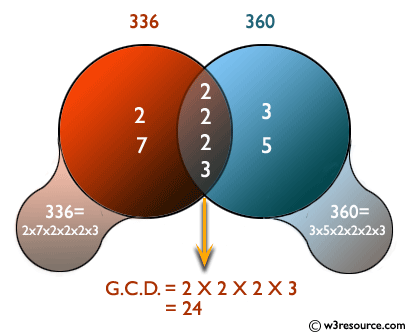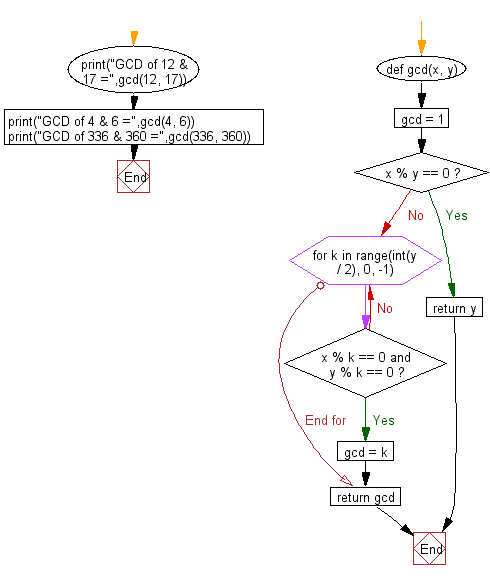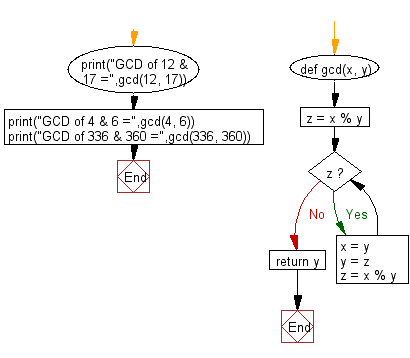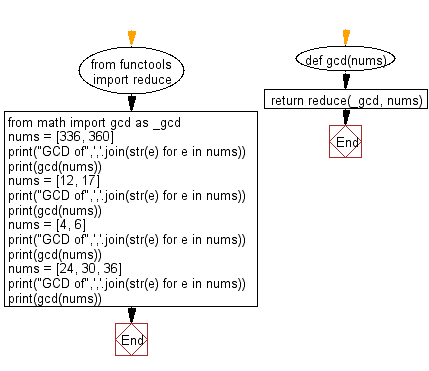﻿ Python: Compute the greatest common divisor (GCD) of two positive integers - w3resource# Python: Compute the greatest common divisor (GCD) of two positive integers

## Python Basic: Exercise-31 with Solution

Write a Python program to compute the greatest common divisor (GCD) of two positive integers.

The greatest common divisor (GCD) of two nonzero integers a and b is the greatest positive integer d such that d is a divisor of both a and b; that is, there are integers e and f such that a = de and b = df, and d is the largest such integer. The GCD of a and b is generally denoted gcd(a, b).

Pictorial Presentation:Sample Solution-1:

Python Code:

``````def gcd(x, y):
gcd = 1
if x % y == 0:
return y
for k in range(int(y / 2), 0, -1):
if x % k == 0 and y % k == 0:
gcd = k
break
return gcd
print("GCD of 12 & 17 =",gcd(12, 17))
print("GCD of 4 & 6 =",gcd(4, 6))
print("GCD of 336 & 360 =",gcd(336, 360))
```
```

Sample Output:

```GCD of 12 & 17 = 1
GCD of 4 & 6 = 2
GCD of 336 & 360 = 24
```

Flowchart:## Visualize Python code execution:

The following tool visualize what the computer is doing step-by-step as it executes the said program:

Sample Solution-2:

Python Code:

``````def gcd(x, y):
z = x % y
while z:
x = y
y = z
z = x % y
return y
print("GCD of 12 & 17 =",gcd(12, 17))
print("GCD of 4 & 6 =",gcd(4, 6))
print("GCD of 336 & 360 =",gcd(336, 360))
```
```

Sample Output:

```GCD of 12 & 17 = 1
GCD of 4 & 6 = 2
GCD of 336 & 360 = 24
```

Flowchart:## Visualize Python code execution:

The following tool visualize what the computer is doing step-by-step as it executes the said program:

Sample Solution-3:

Use functools.reduce() and math.gcd() over the given list.

Python Code:

``````from functools import reduce
from math import gcd as _gcd
def gcd(nums):
return reduce(_gcd, nums)
nums = [336, 360]
print("GCD of",','.join(str(e) for e in nums))
print(gcd(nums))
nums = [12, 17]
print("GCD of",','.join(str(e) for e in nums))
print(gcd(nums))
nums = [4, 6]
print("GCD of",','.join(str(e) for e in nums))
print(gcd(nums))
nums = [24, 30, 36]
print("GCD of",','.join(str(e) for e in nums))
print(gcd(nums))
```
```

Sample Output:

```GCD of 336,360
24
GCD of 12,17
1
GCD of 4,6
2
GCD of 24,30,36
6
```

Flowchart:## Visualize Python code execution:

The following tool visualize what the computer is doing step-by-step as it executes the said program:

Python Code Editor:

Have another way to solve this solution? Contribute your code (and comments) through Disqus.

What is the difficulty level of this exercise?

Test your Python skills with w3resource's quiz

﻿

## Python: Tips of the Day

Try-catch-else construct:

```try:
foo()
except Exception:
print("Exception occured")
else:
print("Exception didnt occur")
finally:
print("Always gets here")
```